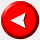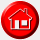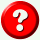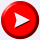# Styles

Diese wunderschöne Überschrift wurde mit der folgender Style-Definition erzeugt:
``````
<h1 style="color:green; font-size:40pt; font-family:Verdana">Styles</h1>
``````

Mit der lokalen Definition
``<p style="text-align:left;margin-left:3cm">``
erreicht man einen linken Rand der Größe 3 cm:

To the left you see a basic Diode Logic OR gate. We'll assume that a logic 1 is represented by +5 volts, and a logic 0 is represented by ground, or zero volts. In this figure, if both inputs are left unconnected or are both at logic 0, output Z will also be held at zero volts by the resistor, and will thus be a logic 0 as well.

Entsprechend erzeugt
``<p style="text-align:right;margin-right:3cm">``
einen rechten Rand der Breit 3 cm.

To the left you see a basic Diode Logic OR gate. We'll assume that a logic 1 is represented by +5 volts, and a logic 0 is represented by ground, or zero volts. In this figure, if both inputs are left unconnected or are both at logic 0, output Z will also be held at zero volts by the resistor, and will thus be a logic 0 as well.